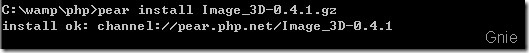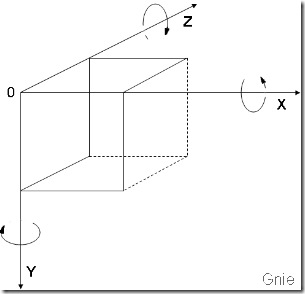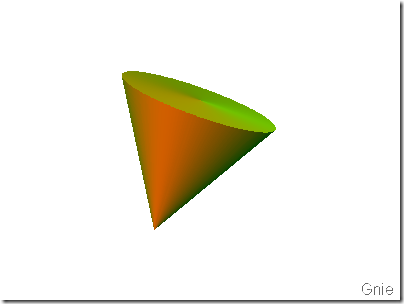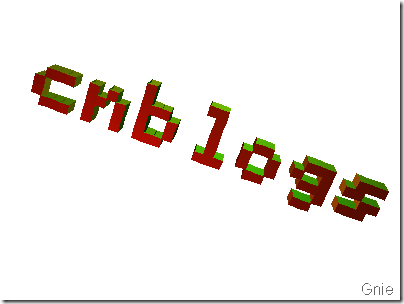# PHP绘制3D图形

PEAR提供了Image_3D Package来创建3D图像。图像或光线在3D空间中按照X、Y 、Z 坐标定位。生成的图像将呈现在2D空间中，可以存储为 PNG、SVG 格式，或输出到Shell。通过Image_3D可以很方便生成一些简单的3D对象，例如立方体、锥体、球体、文本和饼图。查了一下PEAR文档关于Image_3D的实例一个也没有～囧～，只好自己研究一下，将经验分享给大家，请多多拍砖。言归正传，Image_3D需要PEAR和GD(配置php.ini)的支持。

#### 1. 安装Image_3D#### 2. 3D坐标系

Image_3D的坐标系及旋转请参考下图，用于缩放、旋转或移动物体：#### 3. 绘制圆锥体

<?php
//调用3D图形类
require_once('Image/3D.php');

//创建一个三维空间
$image = new Image_3D();$image->setColor(new Image_3D_Color(255, 255, 255));

//在坐标系左下方创建一个红色光点
$light1 =$image->createLight("Light",array(-100, 100, -100));
$light1->setColor(new Image_3D_Color(255, 0, 0)); //在坐标系右上方创建一个绿色光点$light2 = $image->createLight("Light",array(100, -200, -50));$light2->setColor(new Image_3D_Color(0, 200, 0));

//创建3D圆锥体
$cone =$image->createObject('cone',array('detail'=>360));
$cone->setColor(new Image_3D_Color(255, 255, 255)); //按X,Y,Z轴缩放$cone->transform($image->createMatrix('scale', array(80, 150, 80))); //按X,Y,Z轴旋转$cone->transform($image->createMatrix('rotation', array(15, 0, 20))); //按X,Y,Z轴移动$cone->transform($image->createMatrix('move', array(0, -50, 0))); //创建2D输出图像 //渲染效果，Image_3D共提供了种效果：Isometric，Perspectively，Raytrace //参考...\PEAR\Image\3D\Renderer目录$image->createRenderer('perspectively');
//图像驱动，也可以SVG，ASCII等
//参考...\PEAR\Image\3D\Driver目录
$image->createDriver('gd'); //创建输出图片名称及大小$image->render(400, 300, 'object.png');
//显示效果图
echo '<img src="object.png">';
?>#### 4. 3D文字效果

//创建3D字体
$text =$image->createObject('text','cnblogs');
$text->setColor(new Image_3D_Color(255, 255, 255));$text->transform($image->createMatrix('scale', array(10, 10, 20)));$text->transform($image->createMatrix('rotation', array(20, 0, 20)));$text->transform(\$image->createMatrix('move', array(-150, -100, 0)));posted @ 2009-08-27 14:12  Gnie  阅读(4678)  评论(8编辑  收藏  举报